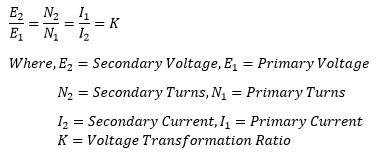Search

# Transformer Winding Resistance

Transformer Winding Resistance

Transformer winding is made of copper or aluminium, and the resistivity of copper and aluminum is 1.68 x 10-8 Ω-m and 2.65 x 10-8 Ω-m respectively. The transformer winding is made of many turns therefore cooper or aluminum is used in meters. The resistance of the conductor is proportional to the length of wire,resistivity and inversely proportional to the area of cross section. Therefore, the primary and the secondary winding of the transformer has finite resistance. In an ideal transformer the winding resistance is considered zero which is totally hypothetical.

The voltage drop in the transformer is caused by voltage drop in resistance and reactance of the transformer. In this section, we are considering only resistance calculation of the transformer. The resistance of the primary and secondary winding as shown below;The winding resistance can be transferred from primary to secondary or vice versa. The voltage drop remains the same either the primary resistance is transferred to secondary side or secondary side resistance is transferred to primary side. When the transformer resistance is referred to one side of the transformer, the calculation of resistance and reactance of transformer becomes very easy.

Before knowing the procedure of how to transfer the resistance from primary to secondary or vice versa, it is essential to understand the voltage transformation ratio of the transformer. The voltage transformation ratio of the transformer is denoted by letter "K'.Case1:

Transformer equivalent resistance when secondary resistance transferred to primary sideLet the R1 and R2 is the resistance of primary and secondary winding of the transformer. I1 and I2 is the primary and secondary current. R’2 is the equivalent resistance of the secondary referred to primary.

When the secondary resistance is referred to primary, the transformer performance parameter like voltage regulation and losses remain the same. The copper loss in secondary winding when I2 current flows through it is given as;Thus, the total resistance referred to primary is;Case2:

Transformer equivalent resistance when primary resistance is transferred to secondary sideLet the R1 and R2 is the resistance of primary and secondary winding of the transformer. I1 and I2 is the primary and secondary current. R’2 is the equivalent resistance of the secondary referred to primary.

When the secondary resistance is referred to primary, the transformer performance parameter like voltage regulation and losses remain the same. The copper loss in secondary winding when I2 current flows through it is given as;Thus, the total resistance referred to secondary is;Related Articles: